# 20 Results

View
Selected filters:
• CCSS.Math.Content.6.G.A.3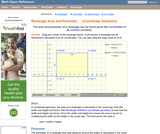Rating

An interactive applet and associated web page showing how to find the area and perimeter of a rectangle from the coordinates of its vertices. The rectangle can be either parallel to the axes or rotated. The grid and coordinates can be turned on and off. The area and perimeter calculation can be turned off to permit class exercises and then turned back on the verify the answers. The applet can be printed as it appears on the screen to make handouts. The web page has a full description of the method for determining area and perimeter, a worked example and has links to other pages relating to coordinate geometry. Applet can be enlarged to full screen size for use with a classroom projector. This resource is a component of the Math Open Reference Interactive Geometry textbook project at http://www.mathopenref.com.

Subject:
Geometry
Material Type:
Simulation
Provider:
Math Open Reference
Author:
John Page
02/16/2011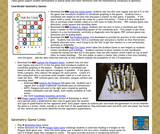Rating

These games support student development of spatial sense and foster familiarity with the mathematical vocabulary of geometry.

Subject:
Geometry
Material Type:
Game
Provider:
Mathwire
Author:
Terry Kawas
02/16/2011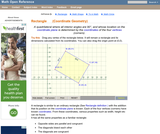Rating

An interactive applet and associated web page that show the definition and properties of a rectangle in coordinate geometry. The applet has a rectangle with draggable vertices. As the user re-sizes the rectangle the applet continuously recalculates its width, height and diagonals from the vertex coordinates. Rectangle can be rotated on the plane to show the more complex cases. The grid, coordinates and calculations can be turned on and off for class problem solving. The applet can be printed in the state it appears on the screen to make handouts. The web page has a full definition of a rectangle when the coordinates of the points defining it are known, and has links to other pages relating to coordinate geometry. Applet can be enlarged to full screen size for use with a classroom projector. This resource is a component of the Math Open Reference Interactive Geometry textbook project at http://www.mathopenref.com.

Subject:
Geometry
Material Type:
Simulation
Provider:
Math Open Reference
Author:
John Page
02/16/2011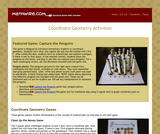Rating

These games support student development of the concept of ordered pairs as they play to win each game.

Subject:
Geometry
Material Type:
Activity/Lab
Game
Provider:
Mathwire
Author:
Terry Kawas
02/16/2011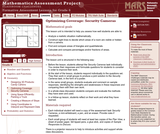Only Sharing Permitted
CC BY-NC-ND
Rating

This lesson unit is intended to help sixth grade teachers assess how well students are able to: Analyze a realistic situation mathematically; construct sight lines to decide which areas of a room are visible or hidden from a camera; find and compare areas of triangles and quadrilaterals; and calculate and compare percentages and/or fractions of areas.

Subject:
Geometry
Ratios and Proportions
Material Type:
Assessment
Lesson Plan
Provider:
Shell Center for Mathematical Education
U.C. Berkeley
Provider Set:
Mathematics Assessment Project (MAP)
04/26/2013Conditional Remix & Share Permitted
CC BY-NC
Rating

Students revise their work on the assessment task based on feedback from the teacher and their peers.Key ConceptsConcepts from previous lessons are integrated into this assessment task: the opposite of a number, integers, absolute value, and graphing points on the coordinate plane. Students apply their knowledge, review their work, and make revisions based on feedback from the teacher and their peers. This process creates a deeper understanding of the concepts.Goals and Learning ObjectivesApply knowledge of the opposite of a number, integers, absolute value, and graphing points on the coordinate plane to solve problems.Track and review a choice of strategy when problem solving.

Subject:
Numbers and Operations
Material Type:
Lesson Plan
Provider:
Pearson
09/21/2015Rating

Surface Area and Volume

Type of Unit: Conceptual

Prior Knowledge

Students should be able to:

Identify rectangles, parallelograms, trapezoids, and triangles and their bases and heights.
Identify cubes, rectangular prisms, and pyramids and their faces, edges, and vertices.
Understand that area of a 2-D figure is a measure of the figure's surface and that it is measured in square units.
Understand volume of a 3-D figure is a measure of the space the figure occupies and is measured in cubic units.

Lesson Flow

The unit begins with an exploratory lesson about the volumes of containers. Then in Lessons 2–5, students investigate areas of 2-D figures. To find the area of a parallelogram, students consider how it can be rearranged to form a rectangle. To find the area of a trapezoid, students think about how two copies of the trapezoid can be put together to form a parallelogram. To find the area of a triangle, students consider how two copies of the triangle can be put together to form a parallelogram. By sketching and analyzing several parallelograms, trapezoids, and triangles, students develop area formulas for these figures. Students then find areas of composite figures by decomposing them into familiar figures. In the last lesson on area, students estimate the area of an irregular figure by overlaying it with a grid. In Lesson 6, the focus shifts to 3-D figures. Students build rectangular prisms from unit cubes and develop a formula for finding the volume of any rectangular prism. In Lesson 7, students analyze and create nets for prisms. In Lesson 8, students compare a cube to a square pyramid with the same base and height as the cube. They consider the number of faces, edges, and vertices, as well as the surface area and volume. In Lesson 9, students use their knowledge of volume, area, and linear measurements to solve a packing problem.

Subject:
Mathematics
Geometry
Material Type:
Unit of Study
Provider:
PearsonOnly Sharing Permitted
CC BY-NC-ND
Rating

This lesson unit is intended to help you assess how well students are able to: Perform arithmetic operations, including those involving whole-number exponents, recognizing and applying the conventional order of operations; Write and evaluate numerical expressions from diagrammatic representations and be able to identify equivalent expressions; apply the distributive and commutative properties appropriately; and use the method for finding areas of compound rectangles.

Subject:
Geometry
Material Type:
Assessment
Lesson Plan
Provider:
Shell Center for Mathematical Education
U.C. Berkeley
Provider Set:
Mathematics Assessment Project (MAP)
04/26/2013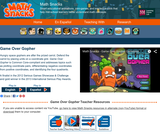Rating

Game Over Gopher is an exciting tower defense game that guides students in plotting coordinate pairs, differentiating negative coordinates from positive coordinates, and identifying the four quadrants. Hungry space gophers are marching towards a prize carrot, and to defend it players place tools around the coordinate grid to “feed” gophers and make them lose interest. Ruby mines (which must be placed at designated x, y coordinates) yield currency that players spend to strategically place carrot launchers, garlic rays, corn silos, and beet traps – gopher feeding tools. To introduce students to the coordinate grid, Game Over Gopher introduces new skills via interactive tutorials and uses consistent visual clues (e.g., x red, y blue) to guide players in plotting coordinates. This means it’s not necessary to teach students coordinate plotting ahead of time. As the levels progress, the number of tools available increases, the level of math vocabulary increases, the scale of the grid changes, and players are asked to expand their mastery of the grid by reflecting points across axes. The game lowers intimidation about the coordinate grid, helps students understand how positive and negative numbers reflect each other across the axes, and helps students get comfortable with the four quadrants.

Subject:
Mathematics
Material Type:
Game
Interactive
Provider:
Learning Games Lab
Author:
NMSU Learning Games Lab
07/20/2015Conditional Remix & Share Permitted
CC BY-NC
Rating

Subject:
Mathematics
Material Type:
Full Course
Provider:
Pearson
10/06/2016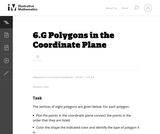Unrestricted Use
CC BY
Rating

This is a task from the Illustrative Mathematics website that is one part of a complete illustration of the standard to which it is aligned. Each task has at least one solution and some commentary that addresses important asects of the task and its potential use. Here are the first few lines of the commentary for this task: The vertices of eight polygons are given below. For each polygon: * Plot the points in the coordinate plane connect the points in the order that they a...

Subject:
Mathematics
Material Type:
Activity/Lab
Provider:
Illustrative Mathematics
Provider Set:
Illustrative Mathematics
Author:
Illustrative Mathematics
09/08/2013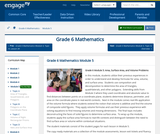Conditional Remix & Share Permitted
CC BY-NC-SA
Rating

In this module, students utilize their previous experiences in order to understand and develop formulas for area, volume, and surface area.  Students use composition and decomposition to determine the area of triangles, quadrilaterals, and other polygons.  Extending skills from Module 3 where they used coordinates and absolute value to find distances between points on a coordinate plane, students determine distance, perimeter, and area on the coordinate plane in real-world contexts.  Next in the module comes real-life application of the volume formula where students extend the notion that volume is additive and find the volume of composite solid figures.  They apply volume formulas and use their previous experience with solving equations to find missing volumes and missing dimensions.  The final topic includes deconstructing the faces of solid figures to determine surface area.  To wrap up the module, students apply the surface area formula to real-life contexts and distinguish between the need to find surface area or volume within contextual situations.

Subject:
Geometry
Material Type:
Module
Provider:
New York State Education Department
Provider Set:
EngageNY
01/31/2014Conditional Remix & Share Permitted
CC BY-NC
Rating

Students play a game in which they try to find dinosaur bones in an archaeological dig simulator. The players guess where the bones are on the coordinate plane using hints and reasoning.Key ConceptsThe coordinate plane consists of a horizontal number line and a vertical number line that intersect at right angles. The point of intersection is the origin, or (0,0). The horizontal number line is often called the x-axis. The vertical number line is often called the y-axis.A point’s location on the coordinate plane can be described using words or numbers. Ordered pairs name locations on the coordinate plane. To find the location of the ordered pair (m,n), first locate m on the x-axis and draw a vertical line through this point. Then locate n on the y-axis and draw a horizontal line through this point. The intersection of these lines is the location of (m,n).The coordinate plane is divided into four quadrants:Quadrant I: (+,+)Quadrant II: (−,+)Quadrant III: (−,−)Quadrant IV: (+,−)Goals and Learning ObjectivesName locations on the coordinate plane.

Subject:
Numbers and Operations
Material Type:
Lesson Plan
Provider:
Pearson
09/21/2015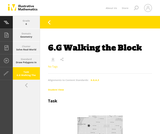Unrestricted Use
CC BY
Rating

This is a task from the Illustrative Mathematics website that is one part of a complete illustration of the standard to which it is aligned. Each task has at least one solution and some commentary that addresses important aspects of the task and its potential use.

Subject:
Geometry
Material Type:
Activity/Lab
Provider:
Illustrative Mathematics
Provider Set:
Illustrative Mathematics
Author:
Illustrative Mathematics
08/06/2015Conditional Remix & Share Permitted
CC BY-NC
Rating

Students reflect a figure across one of the axes on the coordinate plane and name the vertices of the reflection. As they are working, students look for and make use of structure to identify a convention for naming the coordinates of the reflected figure.Key ConceptsWhen point (m,n) is reflected across the y-axis, the reflected point is (−m,n).When point (m,n) is reflected across the x-axis, the reflected point is (m,−n).When point (m,n) is reflected across the origin (0,0), the reflected point is (−m,−n).Goals and Learning ObjectivesReflect a figure across one of the axes on the coordinate plane.Name the vertices of the reflected figure.Discern a pattern in the coordinates of the reflected figure.

Subject:
Numbers and Operations
Material Type:
Lesson Plan
Provider:
Pearson
09/21/2015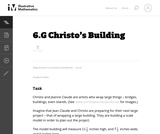Unrestricted Use
CC BY
Rating

This task is primarily about volume and surface area, although it also gives students an early look at converting between measurements in scale models and the real objects they correspond to.

Subject:
Mathematics
Geometry
Material Type:
Activity/Lab
Provider:
Illustrative Mathematics
Provider Set:
Illustrative Mathematics
Author:
Illustrative Mathematics
05/01/2012Only Sharing Permitted
CC BY-NC-ND
Rating

This lesson unit is intended to help teachers assess how well students are able to: Select appropriate mathematical methods to use for an unstructured problem; interpret a problem situation, identifying constraints and variables, and specify assumptions; work with 2- and 3-dimensional shapes to solve a problem involving capacity and surface area; and communicate their reasoning clearly.

Subject:
Geometry
Material Type:
Assessment
Lesson Plan
Provider:
Shell Center for Mathematical Education
U.C. Berkeley
Provider Set:
Mathematics Assessment Project (MAP)
04/26/2013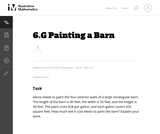Unrestricted Use
CC BY
Rating

The purpose of this task is to provide students an opportunity to use mathematics addressed in different standards in the same problem.

Subject:
Mathematics
Geometry
Material Type:
Activity/Lab
Provider:
Illustrative Mathematics
Provider Set:
Illustrative Mathematics
Author:
Illustrative Mathematics
05/01/2012Conditional Remix & Share Permitted
CC BY-NC
Rating

Students draw a figure on the coordinate plane that matches a written description.Key ConceptsOrdered pairs name locations on the coordinate plane. The first coordinate tells how many units to go left or right of the origin (0,0) along the x-axis. The second coordinate tells how many units to go up or down from the origin along the y-axis.Goals and Learning ObjectivesDraw a figure that matches a description of a figure on the coordinate plane.Give coordinates of points on the coordinate plane.Write descriptions of figures on the coordinate plane.

Subject:
Numbers and Operations
Material Type:
Lesson Plan
Provider:
Pearson
09/21/2015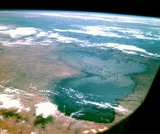Conditional Remix & Share Permitted
CC BY-NC
Rating

Lesson OverviewStudents estimate the area of Lake Chad by overlaying a grid on the map of the lake.Key ConceptThe area of an irregular figure can be found by overlaying a grid on the figure. By estimating the number of grid squares the figure covers and multiplying by the area of each square, you can find the approximate area of the figure. The accuracy of the estimate depends on the size of the grid squares. Using a smaller grid leads to a more accurate estimate because more whole grid squares are completely filled. However, using a smaller grid also requires more counting and more combining of partially-filled squares and is, therefore, more time-consuming. Using a larger grid gives a quicker, but rougher, estimate of the area.Goals and Learning ObjectivesUse a grid to find the area of an irregular figure.MaterialsMap of Lake Chad handout (one for each pair of students)Rulers, optional (one for each pair of students)

Subject:
Geometry
Material Type:
Lesson Plan
Provider:
Pearson